### Home > MC2 > Chapter Ch9 > Lesson 9.2.2 > Problem9-52

9-52.
1. ΔABC and ΔDEF are similar. Homework Help ✎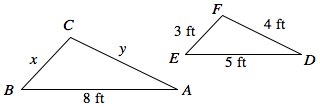1. Find x.

2. Find y.

3. Find the ratio of the perimeters of the two triangles.

4. Can you predict the ratio of the areas of the two triangles? If so, state it. If not, write the information you would need or the question you would need to answer first.

5. Triangle RST is congruent to triangle DEF. Based on this, what are the side lengths of triangle RST? What is the measure of angle E?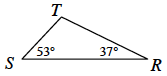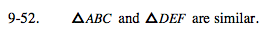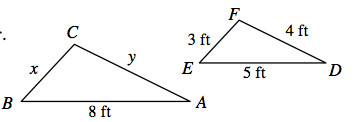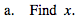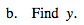Find the scale factor between the two corresponding sides that are given and utilize it with the respective sides from
triangle DEF to get x and y.

$\text{The scale factor is }\frac{8}{5}$

$x = \frac{24}{5}$

$y = \frac{32}{5}$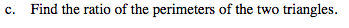Find the perimeter of both triangles and compare them as a ratio. What do you notice?

Perimeter (ABC): 19.2
Perimeter (DEF): 12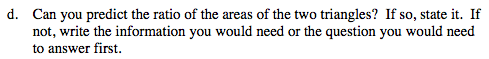According to the Math Notes box from Lesson 9.2.2: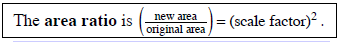$\left( \frac{8}{5} \right)^2 = \frac{64}{25}$

Try predicting the area ratio of the two triangles. What do you get?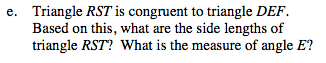Examine the two triangles and the corresponding matching sides or angles to determine each measure.